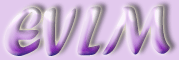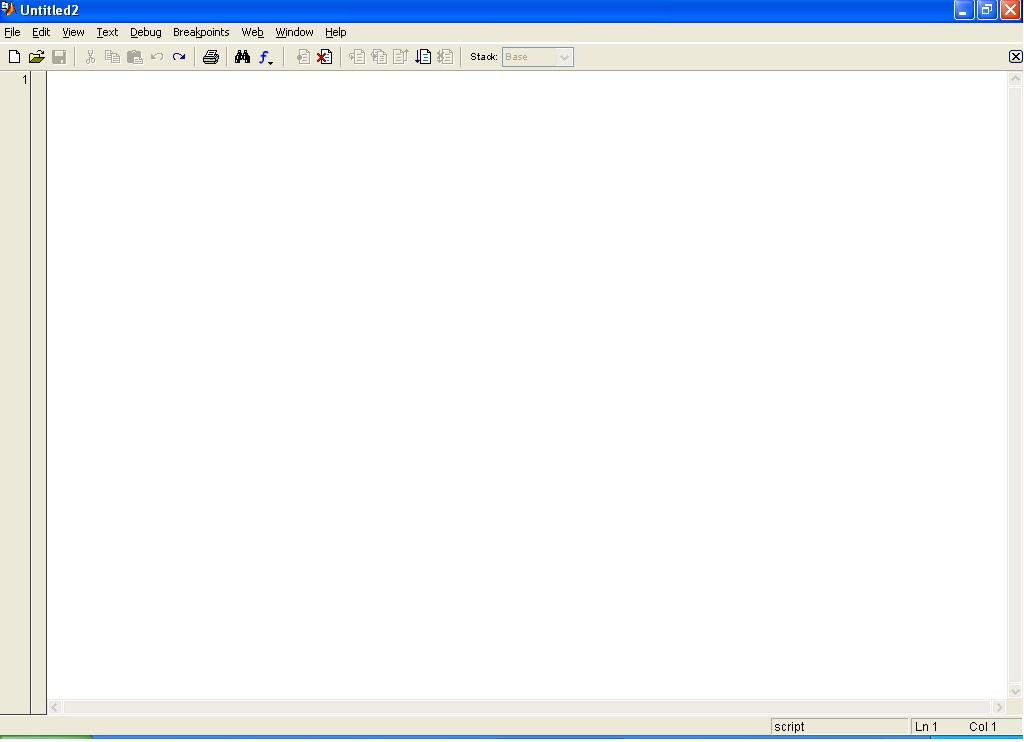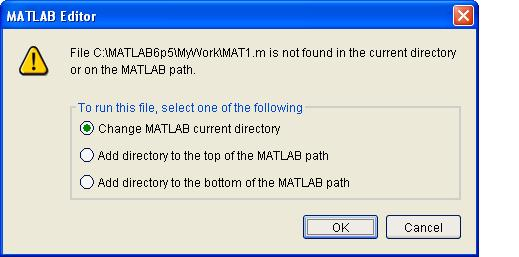Writing M-Files in MATLAB

# 1 What is an M-File?

Although MATLAB can be used as a very sophisticated calculator, its true power lies in its ability to read and execute files written by the user. These are called M-Files, and have a file extension .m . It is good practice to write commands directly into an M-File, and save this to a suitable directory. If an error is made, it is much easier to edit the file than to keep scrolling through various commands, and additionally, an M-File can be saved then subsequently retrieved at a later date. There are two fundamentally different types of M-File, the script file and the function file. A script file contains a sequence of MATLAB commands which are executed when the name of the file is entered into the command window. A function file requires an input, which it then processes and returns as output. MATLAB contains an enormous number of inbuilt function files. These range from the elementary (e.g. 'sin', 'exp') to a large number of specialist functions. All function files are characterised by their ability to take an input from the command line and return an output to the command window without the requirement for editing the function file. A simple example of a function file is given by the exponential function i.e. $\mathrm{exp}\left(x\right)={e}^{x}$. Enter

y=exp(1)

giving the output

y=2.7183

Entering

y=exp(0)

gives the output

y=1

# 2 How to create and use an M-File

## 2.1 Creating a personal folder

First you will need to create a folder in which to deposit your M-File. Find a suitable directory from the "Current Directory" drop down box at the top of the MATLAB window. In the Current Directory window (top left), press the "New Folder" button (shown as a sparkling yellow folder). Give the new folder a suitable name e.g. "MyWork".

## 2.2 Creating an M-file

The fastest way to create an M-File is to click on the "New M-File" button situated at the top left-hand corner of the MATLAB screen, underneath the "File" button. Alternatively, pressing "File - New - M-File" gives the same result. This produces a window similar to the one shown here:As a simple example, open an M-File in MATLAB, then save the M-File as a name of your choice, say, "MAT1.m", to the folder you have just created. It is good practice to make sure that you do not attempt to duplicate the name of an existing M-File! An easy way to check is by entering the command

help MAT1

at the command line window. This returns

Contrast this with the command

help sin

This returns

SIN Sine.

SIN(X) is the sine of the elements of X.

help sym/sin.m

indicating that there is already in existence an M-File called "sin.m".

## 2.3 Creating a script file

We will create a very simple script file to perform the addition of two numbers. At line 1 of your newly created file, input

y=3+2

(note that ";" is not inserted, otherwise we cannot view the output) then press "File - Save As" and save the file as MAT1 to your folder. Press the "Debug - Run" button. You should see this:Pressing the "OK" button allows your folder to be recognised by MATLAB. If you now enter the command

MAT1

y=5

which is what we would hope. If you instead see

??? Undefined function or variable 'MAT1'.

this means that you failed to press the "OK" button in the MATLAB Editor window.

If any changes need to be made to the input, the file has to been opened and edited. For example, if we want to change MAT1 to calculate 3+3, the file content must be changed to

y=3+3

followed by saving the file. Entering MAT1 in the command window now gives us the response

y=6

It is good practice to enter a few comments into each M-file explaining what the file actually does. These are generally entered at the top of the file. It is easy enough to edit your file, simply by clicking on the top left hand point, then, by pressing $\left[$ the first line of code is moved to the next line. Each line of comments must commence with a "%". This tells MATLAB to ignore the line and look for the next line of code which does not commence with "%". In the first line of your M-file, insert the comment

%MAT1 - Calculates the sum of two numbers

Note that MATLAB automatically changes the font colour to green. This is to facilitate easy visual debugging. If we run the file, we get the same answer as before. If, however, you have forgotten to start the first line with "%", MATLAB gives the response

??? Error: File: add.m Line: 2 Column: 18

Unexpected MATLAB expression .

and does not carry out the computation.

## 2.4 Creating a function file

By contrast, we will now create a function file to add two numbers. Open another M-File. Save this as add to your folder (N.B. an existing M-File called 'add' does not exist!). Now enter the following:

%ADD - Calculates the sum of two numbers

out=x+y;

and save the file. Notice the convention of placing "function [out] = add(x,y)" on the first line, followed by the comment on the second line. Now enter